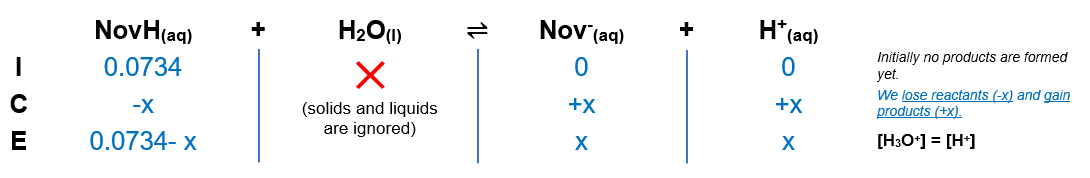# Problem: Novocaine, C13H21O2N2Cl, is the salt of the base procaine and hydrochloric acid. The ionization constant for procaine is 7 × 10−6. Is a solution of novocaine acidic or basic? What are [H3O+], [OH−], and pH of a 2.0% solution by mass of novocaine, assuming that the density of the solution is 1.0 g/mL.

###### FREE Expert Solution

(1) Ka of novocaine

Ka = 1.4x10-8

(2) Calculate molarity of novocaine

M = 0.0734 M

(3) Construct an ICE chart(4) Calculate x###### Problem Details

Novocaine, C13H21O2N2Cl, is the salt of the base procaine and hydrochloric acid. The ionization constant for procaine is 7 × 10−6. Is a solution of novocaine acidic or basic? What are [H3O+], [OH], and pH of a 2.0% solution by mass of novocaine, assuming that the density of the solution is 1.0 g/mL.